Next: Factorization of Spinor-Wavefunctions Up: Spin Angular Momentum Previous: Pauli Two-Component Formalism

# Spinor Rotation Matrices

A general rotation operator in spin space is written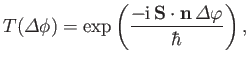(5.95)

by analogy with Equation (5.24), where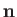is a unit vector pointing along the axis of rotation, and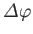is the angle of rotation. Here,can be regarded as a trivial position operator. The rotation operator is represented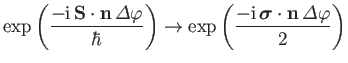(5.96)

in the Pauli scheme. The term on the right-hand side of the previous expression is the exponential of a matrix. This can easily be evaluated using the Taylor series for an exponential, plus the rules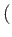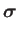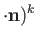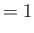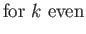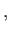(5.97)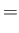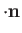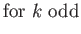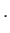(5.98)

These rules follow trivially from the identity (5.93). Thus, we can write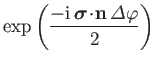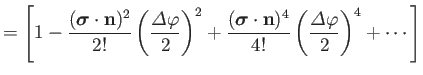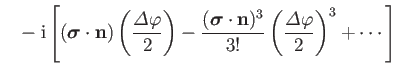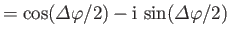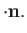(5.99)

The explicit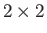form of this matrix is(5.100)

Rotation matrices act on spinors in much the same manner as the corresponding rotation operators act on state kets. Thus,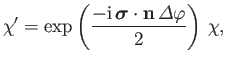(5.101)

where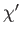denotes the spinor obtained after rotating the spinor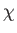an angleabout the axis. The Pauli matrices remain unchanged under rotations. However, the quantity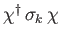is proportional to the expectation value of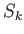[see Equation (5.75)], so we would expect it to transform like a vector under rotation. (See Section 5.4.) In fact, we require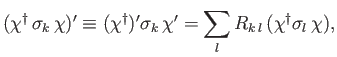(5.102)

where the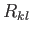are the elements of a conventional rotation matrix . This is easily demonstrated, because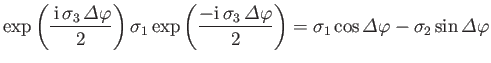(5.103)

plus all cyclic permutations. The previous expression is thematrix analog of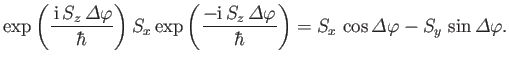(5.104)

[See Equation (5.30).] Equation (5.103) follows from the Baker-Campbell-Hausdorff lemma, (5.31), which holds for matrices, in addition to operators.Next: Factorization of Spinor-Wavefunctions Up: Spin Angular Momentum Previous: Pauli Two-Component Formalism
Richard Fitzpatrick 2016-01-22